Function Repository Resource:

# TuringMachineFromNumber

Convert numbers specifying a Turing machine into an explicit list of rules

Contributed by: Jan Mangaldan and Mano Namuduri (original content by Stephen Wolfram)
 ResourceFunction["TuringMachineFromNumber"][n,s,k] converts a Turing machine specified with rule number n,s states and k colors into an equivalent List of rules. ResourceFunction["TuringMachineFromNumber"][n,s] converts a Turing machine specified with rule number n,s states and two colors into an equivalent List of rules. ResourceFunction["TuringMachineFromNumber"][n] converts a Turing machine specified with rule number n, two states and two colors into an equivalent List of rules.

## Details

The number of possible Turing machine rules is as follows:
 2-state, 2-color machines 4096 s-state, k-color machines (2 s k)s k

## Examples

### Basic Examples (2)

Generate the List of rules corresponding to 2-state, 2-color machine 2506:

 In:=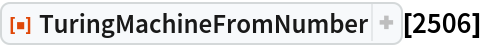Out=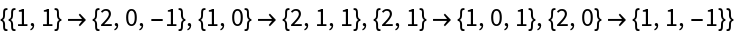Show that the two specifications are equivalent:

 In:=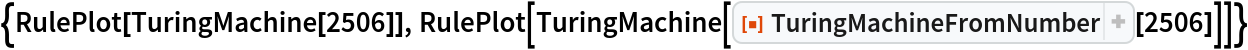Out=### Scope (3)

Generate the rule representation of Wolfram’s simplest universal Turing machine:

 In:=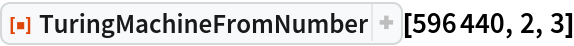Out=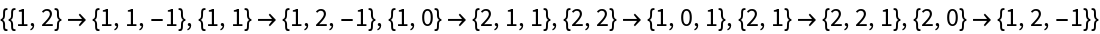Generate the rule representation of a 3-state, 2-color machine:

 In:=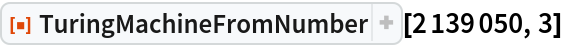Out=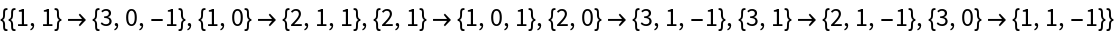Generate the rule representation of a 2-state, 2-color machine:

 In:=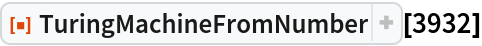Out=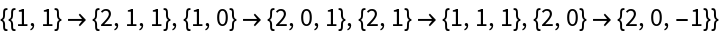### Possible Issues (1)

Supplying a rule number outside the allowed range imposed by the state and color specifications results in an error:

 In:=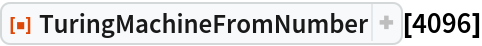Out=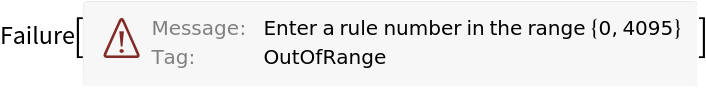## Version History

• 2.0.0 – 05 April 2021
• 1.0.0 – 21 September 2020